DG7YBN - Low Noise Yagis

DG7YBN
Low Noise Yagis

DG7YBN
Low Noise Yagis

Online Calculators
(x)

# RF Calculators

Last Update Oct. 24th 2021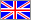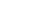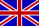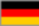General Issues

EIRP : Equivalent isotropically radiated power

EIRP Calc.

 Watts at Ant. Ant. Gain [dBi]

 EIRP in W and kW

EIRP is a term often used with the topic of RFI (Radio-Frequency Interference) and Radiation Power of Electromagnetic Fields. Simply put EIRP stands for the equivalent of fed power to an isotropic radiator (gain = 0 dBi per definition) vs. a directional antenna with certain gain over the iso.-radiator and other fed power. By referring to the iso.-radiator directional power levels in beam direction can easily be compared. In Amateur Radio use that could be comparing powers of two stations. Lets assume a DX setup for 432 MHz: one station is feeding 700 W into a 17.1 dBi antenna, the other 400 W into a 19.8 dBi antenna. Which one 'pumps' more radiation power into beam direction?

What maths are driving this calculator?

1. Conversion of Isotropic Gain of Antenna to numeric number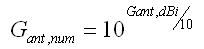2. EIRP is derived by multiplication of Power in watts with numeric Gain of antenna against an Isotropic Radiator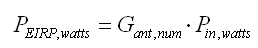'Gain,iso' = dBi = dBd + 2.15 dB. Example: 12.10 dBd is 14.25 dBi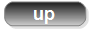Watts to dBm & dBm to watts Conversion Calculator

W > dBm
 W   > dBm

dBm > W
 dBm   > W

dBm is Decibel-milliwatt, it references power to one mW - with 0 dBm = 1 mW

•    0 dBm = 0.001 W
•  10 dBm =   0.01 W
•  50 dBm =   100 W

What maths are driving this calculator?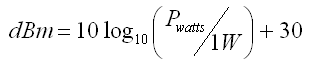dB Conversion Calculator

Gain, numeric > dB
 Gain [numeric]   > Gain [dB]

Gain, dB > numeric
 Gain [dB]   > Gain [numeric]

This conversion works with GAIN and LOSSES:
• Gain  3.0 dB = 2.00 numeric
• Gain     0 dB = 1.00 numeric
• Gain -3.0 dB = 0.50 numeric

What maths are driving this calculator?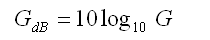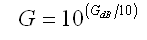Getting a sense for numeric gain numbers as Power in Watts is multiplied with numeric Gain, see EIRP Calculator:
• 2.00 numeric = we know that 3 dB gain gives double output
• 1.00 numeric = adding 0 dB does not change anything
• 0.50 numeric = is half power outAmplification Gain or Attenuation to Watts in/out Calculator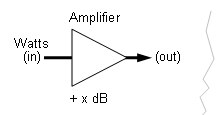Watts in, Gain or Loss to Watts out

 Watts input   > Gain/(-)Loss [dB]

 Watts out [W]

What maths are driving this calculator?

Power Levels in dB can be summed (gain) or subtracted (loss).
So we convert Power Input in watts to Power Level (dB) refering to 1 watt (dBw)Next we add the Power Levels of fed power and gain or attenuation block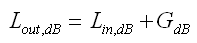or generalised for any chain of gain and attenuation blocks the formula looks like this:
Note the rather explicite notation 'Loss_x'. This commonly would be another G_x with gain < 1.00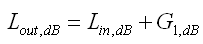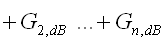Finally we convert the resulting Power Level (dB) into watts again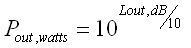Temperature to dB Calculator

Kelvin to dB Calculator

 Kelvin [K]

Computed dB or NF

What maths are driving this calculator?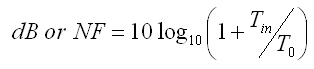with To = 290 K = 17° Celsius mean ambient temp. (273 K = 0° C)And in reverse ... dB to Kelvin Calculator

dB to Kelvin Calculator

 dB or NF

Computed Temp. [K]

What maths are driving this calculator?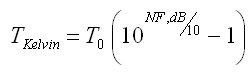with To = 290 K = 17° Celsius mean ambient temp. (273 K = 0° C)μV to dBm Calculator

μV to dBm Calculator

 μV ohms

Computed dBm

What maths are driving this calculator?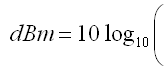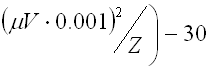dBm is Power Level referring to 1 mWdBm to NF Calculator      Receiver Sensitivity to Noise Figure

dBm to NF Calculator

 dBm BW (kHz) S/N (dB)

Computed dB or NF

• BW = Bandwidth
• dBm = Power Level referring to 1 mW
• To = 290 K or 17° C ambient

Using this calculator with Receiver Specs from a Data Sheets

As different S/N levels are used as well as bandwidth depends, look up those specifications
with the μV or dBm number given for sensitivity and enter to calculator form to yield a correct result

Typical numbers

• nominal BW's: CW or as SSB = from 2.4 ...3 kHz, AM = 6 kHz, FM = 12.5 ... 25 kHz
• CW Filter? => acc. filters bandwidth settings
• nominal S/N = 6, 10 or 12 dB

What maths are driving this calculator?

k = Boltzman's constant (1.3 x 10-23 J/K)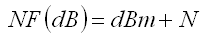SNR to S-Units and μV Calculator

Enter in dBm

IARU Region 1 rec. for Base Level
Short Wave = -121 dBm, VHF and up: -141 dBm

 Base level Noise Floor Signal

 SNR   dB S-Units, absolute    /    plus dB S-Units, relative to noise floor    /    plus dB Volts at 50 ohms   μVG/T Calculator

G/T - Ratio

 Gain (dBi) Temp. (K)

Computed G/T (dB)

What maths are driving this calculator?VSWR & Mismatch Loss

Z1 would be Zo or 'at Transmitter or Receiver' while Z2 could be antenna feedpoint impedance

Mismatch Loss (ML) expresses what Losses derive from connecting non similar impedances

VSWR & Mismatch Loss

 Z1(Ω) Z2(Ω) =R + j

 Z2 absolute (Ω) VSWR ( / ) Refl. Coefficient Γ ( / ) Return Loss (dB) Mismatch Loss (dB)

Mismatch Loss application example:

Given a 50 ohms feedline coax of 10 m length with a loss of 1.2 dB shall be connected to a 75 ohms antenna. What is the total loss here?

The calculator puts out -0.177 dB for Mismatch Loss, the coax adds 1.2 dB, total loss is 1.377 dB.

What maths are driving this calculator?

Z2 in absolute numbers is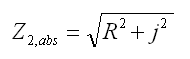with this we do the rest as follows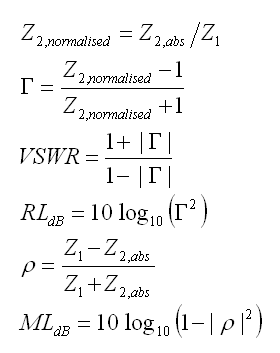73, Hartmut, DG7YBN